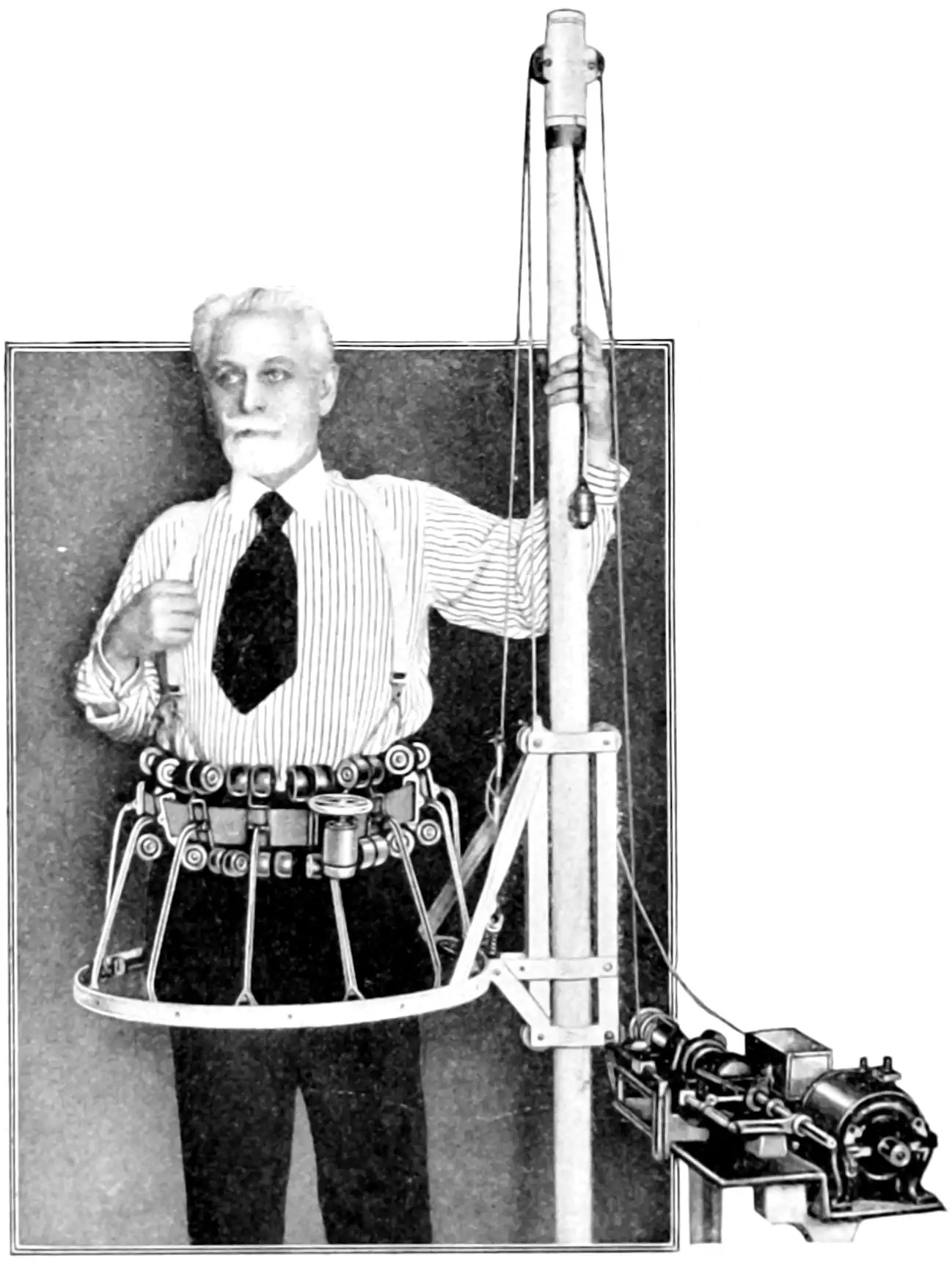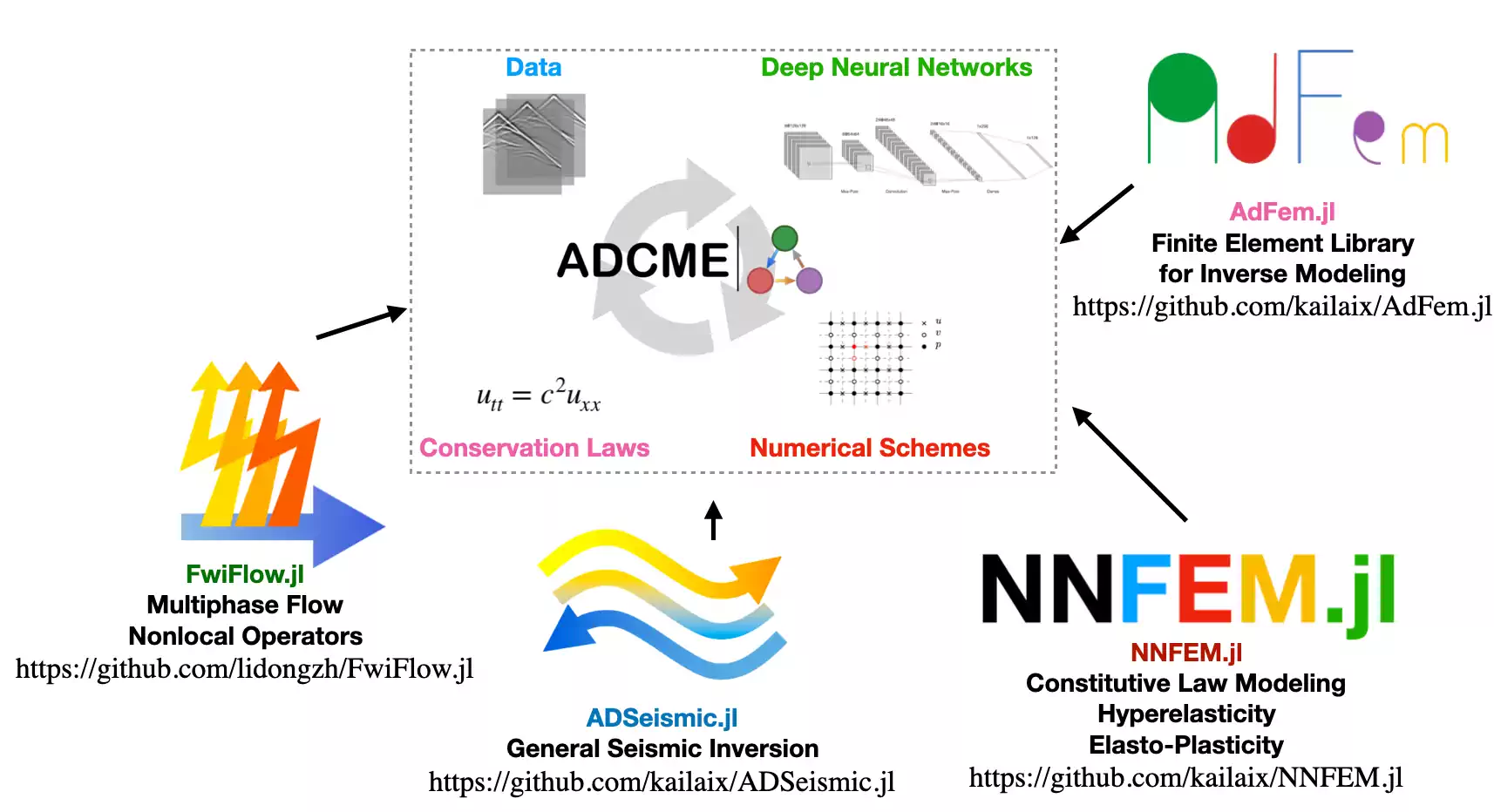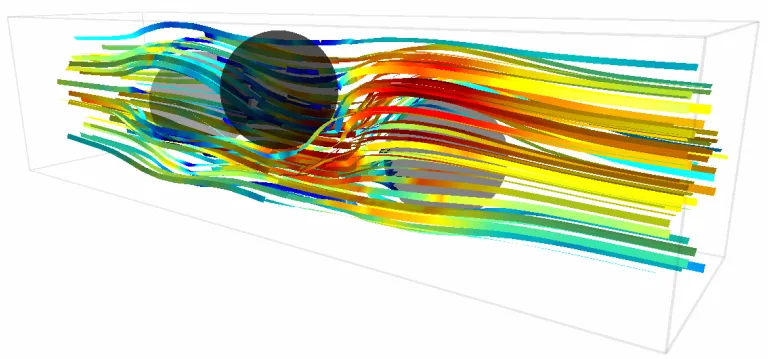# Numerical PDE solversA numerical solver iteratively massages an initial condition into a solution with the required form

There are many methods for numerically solving partial differential equations. Finite element methods, geometric multigrid, algebraic multigrid. Stochastic methods… Building a solver from base principles is a wonderful and improving activity but something that I do not wish to do recreationally. For a list of particular methods designed to be differentiabel in their parameters, see differentiable PDE solvers.

## Dune/Dumux

A porous flow solver, useful in e.g. hydrology.

## Clawpack

Clawpack documentation

Clawpack is a collection of finite volume methods for linear and nonlinear hyperbolic systems of conservation laws. Clawpack employs high-resolution Godunov-type methods with limiters in a general framework applicable to many kinds of waves. Clawpack is written in Fortran and Python.

What my colleagues call a “finite volume” method, AFAICT There is a python… interface? Port?

## Dedelus

Dedalus Project >Dedalus solves differential equations using spectral methods. >It’s open-source, written in Python, and MPI-parallelized. > >We develop and use Dedalus to study fluid dynamics, but it’s designed to solve initial-value, boundary-value, and eigenvalue problems involving nearly arbitrary equations sets. You build a spectrally-representable domain, symbolically specify equations and boundary conditions, select a numerical solver, and go.

If my problem happens to be neatly expressible as a product of This one looks extra nice because sometimes I just want problems solved on a raster grid without all the flexibility of the FEM solvers.

A Julia system using Tensorflow to handle some of the autodiff mechanics.

ADCME is suitable for conducting inverse modeling in scientific computing; specifically, ADCME targets physics informed machine learning, which leverages machine learning techniques to solve challenging scientific computing problems. The purpose of the package is to:

1. provide differentiable programming framework for scientific computing based on TensorFlow automatic differentiation (AD) backend;
2. adapt syntax to facilitate implementing scientific computing, particularly for numerical PDE discretization schemes;
3. supply missing functionalities in the backend (TensorFlow) that are important for engineering, such as sparse linear algebra, constrained optimization, etc.

Applications include

• physics informed machine learning (a.k.a., scientific machine learning, physics informed learning, etc.)
• coupled hydrological and full waveform inversion
• constitutive modeling in solid mechanics
• learning hidden geophysical dynamics
• parameter estimation in stochastic processes

The package inherits the scalability and efficiency from the well-optimized backend TensorFlow. Meanwhile, it provides access to incorporate existing C/C++ codes via the custom operators. For example, some functionalities for sparse matrices are implemented in this way and serve as extendable ”plugins” for ADCME.

AFAICT this implies that GPUs are not in general supported.## JuliaFEM

Julaifem is an umbrella organisation supporting julia-backed FEM solvers. The documentation is tricksy, but check out the examples. Analyses and solvers · JuliaFEM.jl

## Trixi

Trixi.jl

Trixi.jl is a numerical simulation framework for hyperbolic conservation laws written in Julia. A key objective for the framework is to be useful to both scientists and students. Therefore, next to having an extensible design with a fast implementation, Trixi is focused on being easy to use for new or inexperienced users, including the installation and postprocessing procedures.

## Gridap.jl

Gridap seems fresh.

## Firedrake

Firedrake seems popular for Python projects that do not need GPU?

Firedrake is an automated system for the solution of partial differential equations using the finite element method (FEM). Firedrake uses sophisticated code generation to provide mathematicians, scientists, and engineers with a very high productivity way to create sophisticated high performance simulations.

• Expressive specification of any PDE using the Unified Form Language from the FEniCS Project.
• Sophisticated, programmable solvers through seamless coupling with PETSc.…
• Sophisticated automatic optimisation, including sum factorisation for high order elements, and vectorisation.

## FEniCS

Also seems to be a friendly PDE solver. Also lacking in GPU support.

FEniCS is a popular open-source (LGPLv3) computing platform for solving partial differential equations (PDEs). FEniCS enables users to quickly translate scientific models into efficient finite element code. With the high-level Python and C++ interfaces to FEniCS, it is easy to get started, but FEniCS offers also powerful capabilities for more experienced programmers. .FEniCS runs on a multitude of platforms ranging from laptops to high-performance clusters.

As an illustration of how to program a simple PDE model with FEniCS, consider the Stokes equations in variational form:

$\int_{\Omega} \mathrm{grad} \, u : \mathrm{grad} \, v \,\mathrm{d}x \, – \int_{\Omega} p \, \mathrm{div} \, v \,\mathrm{d}x + \int_{\Omega} \mathrm{div} \, u \, q \,\mathrm{d}x = \int_{\Omega} f \cdot v \,\mathrm{d}x.$

The variational problem is easily transcribed into Python using mathematical operators in FEniCS:

# Define function space
P2 = VectorElement('P', tetrahedron, 2)
P1 = FiniteElement('P', tetrahedron, 1)
TH = P2 * P1
W = FunctionSpace(mesh, TH)

# Define variational problem
(u, p) = TrialFunctions(W)
(v, q) = TestFunctions(W)
L = dot(f, v)*dx

# Compute solution
w = Function(W)
solve(a == L, w, [bc1, bc0])Ooh, pretty

## Mantaflow

mantaflow: an extensible framework for fluid simulation

mantaflow is an open-source framework targeted at fluid simulation research in Computer Graphics and Machine Learning. Its parallelized C++ solver core, python scene definition interface and plugin system allow for quickly prototyping and testing new algorithms. A wide range of Navier-Stokes solver variants are included. It’s very versatile, and allows coupling and import/export with deep learning frameworks (e.g., tensorflow via numpy) or standalone compilation as matlab plugin. Mantaflow also serves as the simulation engine in Blender.

Feature list

The framework can be used with or without GUI on Linux, MacOS and Windows. An incomplete list of features implemented so far:

• Eulerian simulation using MAC Grids, PCG pressure solver and MacCormack advection
• Flexible particle systems
• FLIP simulations for liquids
• Surface mesh tracking
• Free surface simulations with levelsets, fast marching
• Wavelet and surface turbulence
• K-epsilon turbulence modeling and synthesis
• Maya and Blender export for rendering

Mantaflow is associated with some projects that examine neural PDE solutions, and also with producing stunning 3d animations as an output.

## scikit-fdidd

Scikit-fdiff is a python library that aim to solve partial derivative equations without pain. As its name says, it uses finite difference method to discretize the spatial derivative. As its name does not say, it is based on method of lines where all the dimension of the PDE but the last (the time) is discretized. That turns the PDE in a high-dimension ODE that can be solved with standard numerical integration of ODEs.

Annoyingly this does not seem to directly support adjoints, but it should not be too hard to hack.

## PolyFEM

PolyFEM is a simple C++ and Python finite element library. We provide a wide set of common PDEs including:

• Laplace
• Helmholtz
• Linear Elasticity
• Saint-Venant Elasticity
• Neo-Hookean Elasticity
• Stokes
• Navier Stokes [beta]

PolyFEM simplicity lies on the interface: just pick a problem, select some boundary condition, and solve. No need to construct complicated function spaces, or learn a new scripting language: everything is set-up trough a JSON interface or through the Setting class in python.

## SfePy

SfePy is a (sic) software for solving systems of coupled partial differential equations (PDEs) by the finite element method in 1D, 2D and 3D. It can be viewed both as black-box PDE solver, and as a Python package which can be used for building custom applications. The word “simple” means that complex FEM problems can be coded very easily and rapidly.

See Cimrman, Lukeš, and Rohan (2019).

## fipy

fipy is original school:

FiPy is an object oriented, partial differential equation (PDE) solver, written in Python, based on a standard finite volume (FV) approach. … The FiPy framework includes terms for transient diffusion, convection and standard sources, enabling the solution of arbitrary combinations of coupled elliptic, hyperbolic and parabolic PDEs. Currently implemented models include phase field treatments of polycrystalline, dendritic, and electrochemical phase transformations as well as a level set treatment of the electrodeposition process.

## PyAMG

pyamg is an algebraic multigrid solver, which is … a kind of multi-resolution method?

AMG is a multilevel technique for solving large-scale linear systems with optimal or near-optimal efficiency. Unlike geometric multigrid, AMG requires little or no geometric information about the underlying problem and develops a sequence of coarser grids directly from the input matrix. This feature is especially important for problems discretised on unstructured meshes and irregular grids:

A = poisson((500,500), format='csr')      # 2D Poisson problem on 500x500 grid
ml = ruge_stuben_solver(A)                # construct the multigrid hierarchy
print ml                                  # print hierarchy information
b = rand(A.shape)                      # pick a random right hand side
x = ml.solve(b, tol=1e-10)                # solve Ax=b to a tolerance of 1e-8
print("residual norm is", norm(b - A*x))  # compute norm of residual vector

See Yousef Saad’s textbooks on algebraic multigrid methods (free online).

## Single-purpose solvers

• nsfds2 is a Navier-Stokes solver in 2d especially for acoustics models

### No comments yet. Why not leave one?

GitHub-flavored Markdown & a sane subset of HTML is supported.